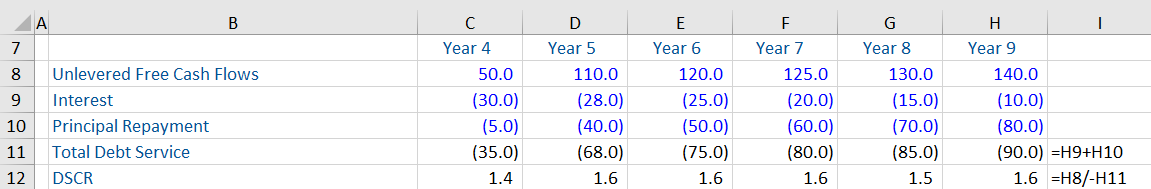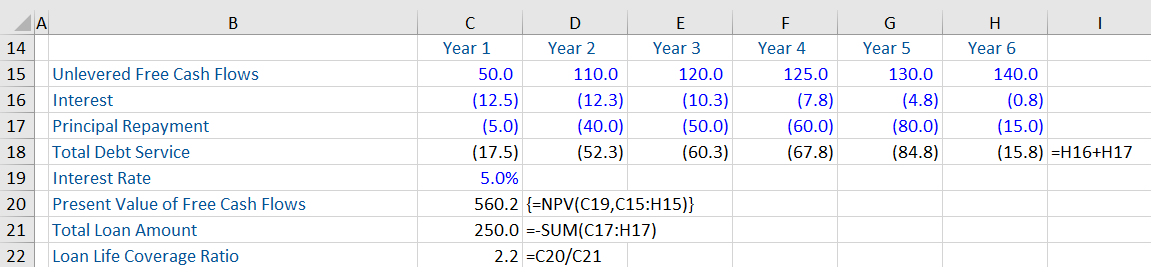## What are “Coverage Ratios?”

Coverage ratios are a set of financial ratios that measure the ability of a company to meet its debt servicing obligations. Such ratios are commonly used by lenders and creditors to analyze a company’s debt servicing ability and financial standing. While leverage ratios focus on the balance sheet of a company, coverage ratios focus on the income statement and cash flows. The common coverage ratios are the debt service coverage ratio (DSCR), loan life coverage ratio (LLCR), interest coverage ratio (ICR), cash coverage ratio (CCR), and the asset coverage ratio (ACR).

## Key Learning Points

• Coverage ratios are commonly used by lenders to assess the debt servicing ability of a company;
• The DSCR and LLCR are typically inserted in loan document and used in project finance; and
• A coverage ratio of more than 1 implies sound ability to meet debt-servicing obligations.

## Coverage Ratios (5) – Formula and Salient Points

The first two coverage ratios (DSCR and LLCR) are typically used in project finance.

The DSCR is calculated as:

DSCR = Unlevered Free Cash Flows/Total Debt Service (each year)

The DSCR compares the unlevered free cash flow (i.e. all the cash available to service debt) of a company with the payment of interest and principal in one year. It measures the cushion a company has over and above the interest and debt repayments.

There is a minimum accepted level of DSCR agreed by the creditors (which may be higher the more risker a project is). For example, if a bank wants a minimum acceptable DSCR of 1.6, this means that for every dollar of interest and principal repayment, the company needs 1.6 dollars of cash flow –  giving 0.6 headroom.

The Loan Life Coverage Ratio (LLCR) is a point in time measure and is calculated as follows:

LLCR = Present Value of Unlevered Free Cash Flows/Total Loan Amount

To compute this ratio, one needs to take the cash flow forecast over the maturity life of the debt. Therefore, if a debt has a maturity of seven years, one needs to take seven years of the cash flow forecast. Then, we take the cash flow forecast and present value it using the cost of debt. Finally, we divide this present value by the amount of the loan to arrive at the LLCR.

If the present value of the cash flows is equal to the loan amount, the LLCR will be one times. Most banks want an LLCR greater than 1, as they want to be covered with some headroom – may be 1.6 or 2 times – depending on the lenders requirements.

The third coverage ratio is the interest coverage ratio that measures the ability of a company to pay the interest expense on its debt. It is computed as:

Interest Coverage Ratio (ICR) = Earnings before Interest and Taxes/Interest Expense

The fourth coverage ratio is the cash coverage ratio (CCR), which is a measure to compare a company’s cash balance to its interest expense (annual). It is computed as:

Cash Coverage Ratio (CCR) = Total Cash/Interest Expense.

The fifth coverage ratio is the asset coverage ratio which measures the ability of a company to meet its debt obligations by selling its assets.

ACR =((Total Assets – Intangible Assets) – (Current Liabilities – Short-Term Debt))/Total Debt Obligations

Usually, a coverage ratio of more than 1 implies that a company is in a sound position to meet its debt related obligations.

## DSCR and LLCR – Examples

In the first example below, we have a cash flow forecasts from year 4 to year 9 and we are assuming that the loan must be paid withing this period. The total debt servicing for each of these years is arrived at by adding the interest expense and repayment of principal each year. Thereafter, the DSCR has been calculated as the unlevered free cash flows of each year divided by total debt service of that particular year. As you can see below, that this ratio improves slightly after year 4 and dips in year 8, and improves again in year 9.In the second example (to calculate the LLCR), we have taken the unlevered free cash flows from year 1 to year 6 and we present value that using the interest rate of 5% to get a net present value (NPV) of 560.2. Thereafter, we divide this value with the original loan amount of 250 to get the LLCR or 2.2.